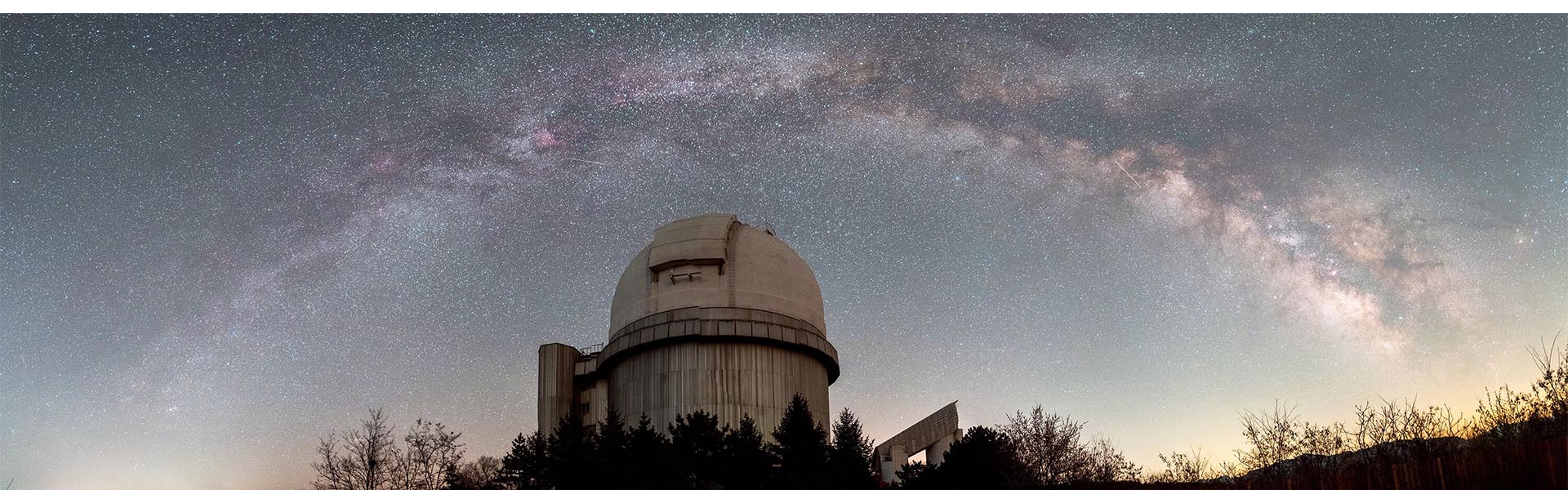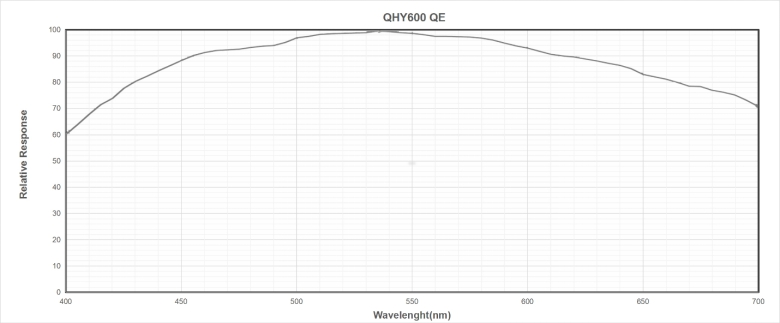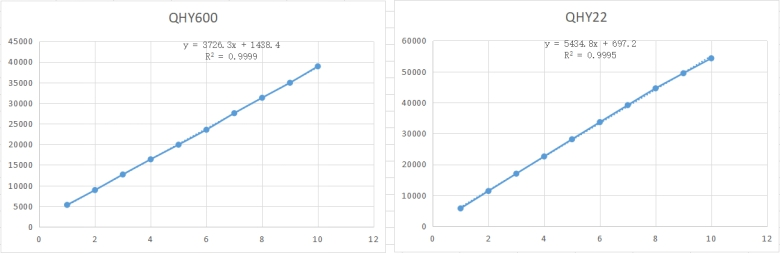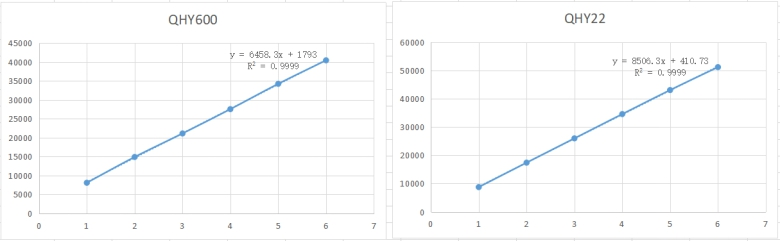HOME > News >
Testing the Absolute QE of the QHY600 BSI CMOS Camera
2019 / 09 / 22
(Preface:  This test is based on a single experiment. The final data looks a little too high and so we plan to find another sensor to do the again. The absolute reference QE of the ICX694 is based on data gathered from others on the internet and there is some variation from different otherwise reliable sources.  We do not know if the QE of the sensor in this particular QHY22 is same as others have tested because each sensor may have a little difference even among sensors of the same model. The system gain of this QHY22 is carefully tested, the ADU per second of the light can be trusted because the linear fitting curve is R^2=0.9999).

The QHY600 uses a SONY IMX455 sensor.  There is an official release of the QE curve for this sensor by Sony.  It is a relative curve and does not indicate the absolute QE.  However, we can determine the actual QE if we can fix one or two points of the curve with reliable absolute data.  Then we can re-map this curve to absolute values.In order to determine the absolute QE data of the QHY600 camera we can compare to a camera with known absolute QE data for a single wavelength.  We use a lens with a narrowband filter to capture an image with each camera against the same place of a uniform flat board, measure the ADUs, then multiply by the system gain to convert the data to photons and then divide by the pixel area of each camera.  In this way we can determine the QE of the QHY600 by comparing to the reference value from the camera with known QE:  Ratio=QE(QHY600)/QE(QHY22) . Then based on the absolute QE curve of the ICX694 sensor we can get the absolute QE of the QHY600 at this wavelength.

In order to avoid differences in any microlens angle effects for wide angle light paths we set the aperture of the lens to F11.We use a 10nm Ha filter and OIII filter.  The reference camera selected in this experiment is QHY22 which uses the SONY ICX694 CCD sensor.  The QE can be found on various websites. We use the data in http://www.astrosurf.com/buil/cameras/index.html as reference data

From this curve we know the absolute QE of ICX694 is 67% at OIII and 58% at Ha.

Test conditions
QHY600 Gain=30 (mode 0) System gain=0.37e/ADU Pixel size=3.76um*3.76um Pixel Area=14.1376um^2
QHY22 Gain=0 System gain=0.29e/ADU Pixel size=4.54um*4.54um Pixel Area=20.6116um^2

Ha Test results with different exposure times
 Exposure QHY600 Measured ADU QHY22 Measured ADU 1S 5305 5753 2S 8895 11373 3S 12682 16977 4S 16355 22551 5S 19903 28112 6S 23534 33651 7s 27542 39147 8s 31282 44574 9s 34925 49457 10s 38910 54291

Using a linear fit we determine the response of the QHY600 is 3726.3 ADU per second and the QHY22 is 5434.8 ADU per second per pixel.Multiple by the system gain: The QHY600 is 1378.6e-/sec/pixel and QHY22 is 1576e-/sec/pixel.

And divide by the pixel area: The QHY600 is 97.51e-/sec/um^2 and QHY22 is 76.47e-/sec/um^2.

It shows on the same exposure and area, for the converted electron, the QHY600 has 1.27 times more than QHY22.  Since the QHY22 QE is 58% at Ha, the QE of QHY600 is 58%*1.27=74%

OIII Test Results with different exposure times

 Exposure QHY600 Measured ADU QHY22 Measured ADU 1s 8109 8776 2s 14916 17421 3s 21131 26011 4s 27550 34597 5s 34233 43091 6s 40443 51201Following the same method as we did for H-alpha we find:

QHY600:  6458.3*0.37/14.1376=169.02e-/sec/um^2
QHY22:   8506.3*0.29/20.6116=119.68e-/sec/um^2

The ratio of QHY600 to QHY22 is 1.41. So, the QE of QHY600 in OIII is 67%*1.41=94.5%

Elsewhere Christian Buil states a lower value of the IMX694 QE at 486nm to be 62% at H-alpha to be 54% (http://www.astrosurf.com/buil/CMOSvsCCD/index.html).  If we substitute these figures as the reference QE, the results for the IMX455 would be 87.4% at OIII and 68.6% at H-alpha.  We therefore conservatively estimate the peak QE of the IMX455 to be greater than 87%.